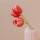Home
IT Knowledge
Inspiration
Languages
EN

# Bash - check if string is empty

0 points
Created by:Pearl-Hurley
369

This article will show you how to check if the string is empty in Bash.

Quick solution:

``````#!/bin/bash

variable="Sample text"
[ -z "\$variable" ] && echo "variable is empty" || echo "variable is not empty"``````

Example output:

``variable is not empty``

## Practical examples

### 1. With `control operators`

``````#!/bin/bash

variable="Sample text"
empty_variable=""

[ -z "\$variable" ] && echo "variable is empty" || echo "variable is not empty"
[ -z "\$empty_variable" ] && echo "empty_variable is empty" || echo "empty_variable is not empty"``````

Example output:

``````variable is not empty
empty_variable is empty``````

### 2. With `if` statement

``````#!/bin/bash

variable="Sample text"

if [ -z "\$variable" ]
then
echo "variable is empty"
else
echo "variable is not empty"
fi``````

Output:

``variable is not empty``

### Alternative `if` statement example

``````#!/bin/bash

variable="Sample text"

if test -z "\$variable"
then
echo "variable is empty"
else
echo "variable is not empty"
fi``````

Output:

``variable is not empty``